• ## CNN的反向传播

千次阅读 2019-03-15 12:48:12
在一般全联接神经网络中，我们通过反向传播算法计算参数导数。BP 算法本质上可以认为是链式法则在矩阵求导上运用。但 CNN卷积操作则不再是全联接形式，因此 CNN BP 算法需要在原始算法上稍作修改...
在一般的全联接神经网络中，我们通过反向传播算法计算参数的导数。BP 算法本质上可以认为是链式法则在矩阵求导上的运用。但 CNN 中的卷积操作则不再是全联接的形式，因此 CNN 的 BP 算法需要在原始的算法上稍作修改。这篇文章主要讲一下 BP 算法在卷积层和 pooling 层上的应用。

原始的 BP 算法

首先，用两个例子回顾一下原始的 BP 算法。（不熟悉 BP 可以参考How the backpropagation algorithm works，不介意的话可以看我的读书笔记）

最简单的例子

先看一个最简单的例子（偷个懒，搬个手绘图～囧～）：上图中，alal 表示第 ll 层的输出（a0a0 就是网络最开始的输入），网络的激活函数假设都是 σ()σ()，wlwl 和 blbl 表示第 ll 层的参数，CC 表示 loss functionloss function，δlδl 表示第 ll 层的误差，zlzl 是第 ll 层神经元的输入，即 zl=wlal−1+blzl=wlal−1+bl，al=σ(zl)al=σ(zl)。

接下来要用 BP 算法求参数的导数 ∂C∂w∂C∂w 和 ∂C∂b∂C∂b。

δ2=∂C∂z2=∂C∂a2∂a2∂z2=∂C∂a2σ′(z2)δ2=∂C∂z2=∂C∂a2∂a2∂z2=∂C∂a2σ′(z2)

δ1=∂C∂z1=δ2∂z2∂a1∂a1∂z1=δ2w2σ′(z1)δ1=∂C∂z1=δ2∂z2∂a1∂a1∂z1=δ2w2σ′(z1)

算出这两个误差项后，就可以直接求出导数了：

∂C∂b2=∂C∂a2∂a2∂z2∂z2∂b2=δ2∂C∂b2=∂C∂a2∂a2∂z2∂z2∂b2=δ2

∂C∂w2=∂C∂a2∂a2∂z2∂z2∂w2=δ2a1∂C∂w2=∂C∂a2∂a2∂z2∂z2∂w2=δ2a1

∂C∂b1∂C∂b1 和 ∂C∂w1∂C∂w1 的求法是一样的，这里不在赘述。

次简单的例子

接下来稍微把网络变复杂一点：符号的标记和上一个例子是一样的。要注意的是，这里的 WlWl 不再是一个数，而变成一个权重矩阵，WlkjWkjl表示第 l−1l−1 层的第 jj 个神经元到第 ll 层的第 kk 个神经元的权值，如下图所示：首先，还是要先求出网络的误差 δδ。

δ21=∂C∂z21=∂C∂a21σ′(z21)δ12=∂C∂z12=∂C∂a12σ′(z12)

δ22=∂C∂z22=∂C∂a22σ′(z22)δ22=∂C∂z22=∂C∂a22σ′(z22)

由此得到：

δ2=[δ21δ22]=⎡⎣⎢∂C∂a21∂C∂a22⎤⎦⎥⊙[σ′(z21)σ′(z22)]δ2=[δ12δ22]=[∂C∂a12∂C∂a22]⊙[σ′(z12)σ′(z22)]

⊙⊙ 表示 elementwise 运算。

接着要根据 δ2δ2 计算前一层的误差 δ1δ1。

δ11=∂C∂z11===∂C∂a21σ′(z21)∂z21∂a11∂a11∂z11+∂C∂a22σ′(z22)∂z22∂a11∂a11∂z11∂C∂a21σ′(z21)W211σ′(z11)+∂C∂a22σ′(z22)W221σ′(z11)[∂C∂a21σ′(z21)∂C∂a22σ′(z22)][W211W221]⊙[σ′(z11)](1)δ11=∂C∂z11=∂C∂a12σ′(z12)∂z12∂a11∂a11∂z11+∂C∂a22σ′(z22)∂z22∂a11∂a11∂z11(1)=∂C∂a12σ′(z12)W112σ′(z11)+∂C∂a22σ′(z22)W212σ′(z11)=[∂C∂a12σ′(z12)∂C∂a22σ′(z22)][W112W212]⊙[σ′(z11)]

同理，δ12=[∂C∂a21σ′(z21)∂C∂a22σ′(z22)][W212W222]⊙[σ′(z12)]δ21=[∂C∂a12σ′(z12)∂C∂a22σ′(z22)][W122W222]⊙[σ′(z21)]。

这样，我们就得到第 1 层的误差项：

δ1=[W211W212W221W222]⎡⎣⎢∂C∂z21∂C∂z22⎤⎦⎥⊙[σ′(z11)σ′(z12)]=W2Tδ2⊙σ′(z1)(2)(2)δ1=[W112W212W122W222][∂C∂z12∂C∂z22]⊙[σ′(z11)σ′(z21)]=W2Tδ2⊙σ′(z1)

然后，根据误差项计算导数：

∂C∂b2j=∂C∂z2j∂z2j∂b2j=δ2j∂C∂w2jk=∂C∂z2j∂z2j∂w2jk=a1kδ2j∂C∂b1j=∂C∂z1j∂z1j∂b1j=δ1j∂C∂w1jk=∂C∂z1j∂z1j∂w1jk=a0kδ1j∂C∂bj2=∂C∂zj2∂zj2∂bj2=δj2∂C∂wjk2=∂C∂zj2∂zj2∂wjk2=ak1δj2∂C∂bj1=∂C∂zj1∂zj1∂bj1=δj1∂C∂wjk1=∂C∂zj1∂zj1∂wjk1=ak0δj1

BP 算法的套路

在 BP 算法中，我们计算的误差项 δlδl 其实就是 loss functionloss function 对 zlzl 的导数 ∂C∂zl∂C∂zl，有了该导数后，根据链式法则就可以比较容易地求出 ∂C∂Wl∂C∂Wl 和 ∂C∂bl∂C∂bl。

CNN 中的 BP 算法

之所以要「啰嗦」地回顾普通的 BP 算法，主要是为了熟悉一下链式法则，因为这一点在理解 CNN 的 BP 算法时尤为重要。

下面就来考虑如何把之前的算法套路用在 CNN 网络中。

CNN 的难点在于卷积层和 pooling 层这两种很特殊的结构，因此下面重点分析这两种结构的 BP 算法如何执行。

卷积层

假设我们要处理如下卷积操作：

⎛⎝⎜a11a21a31a12a22a32a13a23a33⎞⎠⎟∗(w11w21w12w22)=(z11z21z12z22)(a11a12a13a21a22a23a31a32a33)∗(w11w12w21w22)=(z11z12z21z22)

这个操作咋一看完全不同于全联接层的操作，这样，想套一下 BP 算法都不知从哪里入手。但是，如果把卷积操作表示成下面的等式，问题就清晰多了（卷积操作一般是要把卷积核旋转 180 度再相乘的，不过，由于 CNN 中的卷积参数本来就是学出来的，所以旋不旋转，关系其实不大，这里默认不旋转）：

z11=a11w11+a12w12+a21w21+a22w22z12=a12w11+a13w12+a22w21+a23w22z21=a21w11+a22w12+a31w21+a32w22z22=a22w11+a23w12+a32w21+a33w22z11=a11w11+a12w12+a21w21+a22w22z12=a12w11+a13w12+a22w21+a23w22z21=a21w11+a22w12+a31w21+a32w22z22=a22w11+a23w12+a32w21+a33w22

更进一步，我们还可以把上面的等式表示成下图：上图的网络结构中，左边青色的神经元表示 a11a11 到 a33a33，中间橙色的表示 z11z11 到 z22z22。需要注意的是，青色和橙色神经元之间的权值连接用了不同的颜色标出，紫色线表示 w11w11，蓝色线表示 w12w12，依此类推。这样一来，如果你熟悉 BP 链式法则的套路的话，你可能已经懂了卷积层的 BP 是怎么操作的了。因为卷积层其实就是一种特殊的连接层，它是部分连接的，而且参数也是共享的。

假设上图中，zz 这一层神经元是第 ll 层，即 z=zlz=zl，a=al−1a=al−1。同时假设其对应的误差项 δl=∂C∂zlδl=∂C∂zl 我们已经算出来了。下面，按照 BP 的套路，我们要根据 δlδl 计算 δl−1δl−1、∂C∂wl∂C∂wl 和 ∂C∂bl∂C∂bl 。

卷积层的误差项 δl−1δl−1

首先计算 δl−1δl−1。假设上图中的 al−1al−1 是前一层经过某些操作（可能是激活函数，也可能是 pooling 层等，但不管是哪种操作，我们都可以用 σ()σ() 来表示）后得到的响应，即 al−1=σ(zl−1)al−1=σ(zl−1)。那么，根据链式法则：

δl−1=∂C∂zl−1=∂C∂zl∂zl∂al−1∂al−1∂zl−1=δl∂zl∂al−1⊙σ′(zl−1)(3)(3)δl−1=∂C∂zl−1=∂C∂zl∂zl∂al−1∂al−1∂zl−1=δl∂zl∂al−1⊙σ′(zl−1)

对照上面的例子，zl−1zl−1 应该是一个 9 维的向量，所以 σ′(zl−1)σ′(zl−1) 也是一个向量，根据之前 BP 的套路，这里需要 ⊙⊙ 操作。

这里的重点是要计算 ∂zl∂al−1∂zl∂al−1，这也是卷积层区别于全联接层的地方。根据前面展开的卷积操作的等式，这个导数其实比全联接层更容易求。以 al−111a11l−1 和 al−112a12l−1 为例（简洁起见，下面去掉右上角的层数符号 ll）：

∇a11==∂C∂z11∂z11∂a11+∂C∂z12∂z12∂a11+∂C∂z21∂z21∂a11+∂C∂z22∂z22∂a11δ11w11∇a11=∂C∂z11∂z11∂a11+∂C∂z12∂z12∂a11+∂C∂z21∂z21∂a11+∂C∂z22∂z22∂a11=δ11w11

∇a12==∂C∂z11∂z11∂a12+∂C∂z12∂z12∂a12+∂C∂z21∂z21∂a12+∂C∂z22∂z22∂a12δ11w12+δ12w11∇a12=∂C∂z11∂z11∂a12+∂C∂z12∂z12∂a12+∂C∂z21∂z21∂a12+∂C∂z22∂z22∂a12=δ11w12+δ12w11

（∇aij∇aij 表示 ∂C∂aij∂C∂aij。如果这两个例子看不懂，证明对之前 BP 例子中的（1）式理解不够，请先复习普通的 BP 算法。）

其他 ∇aij∇aij 的计算，道理相同。

之后，如果你把所有式子都写出来，就会发现，我们可以用一个卷积运算来计算所有 ∇al−1ij∇aijl−1：

⎛⎝⎜⎜⎜00000δ11δ2100δ12δ2200000⎞⎠⎟⎟⎟∗(w22w12w21w11)=⎛⎝⎜∇a11∇a21∇a31∇a12∇a22∇a32∇a13∇a23∇a33⎞⎠⎟(00000δ11δ1200δ21δ2200000)∗(w22w21w12w11)=(∇a11∇a12∇a13∇a21∇a22∇a23∇a31∇a32∇a33)

这样一来，（3）式可以改写为：

δl−1=∂C∂zl−1=δl∗rot180(Wl)⊙σ′(zl−1)(4)(4)δl−1=∂C∂zl−1=δl∗rot180(Wl)⊙σ′(zl−1)

（4）式就是 CNN 中误差项的计算方法。注意，跟原始的 BP 不同的是，这里需要将后一层的误差 δlδl 写成矩阵的形式，并用 0 填充到合适的维度。而且这里不再是跟矩阵 WlTWlT 相乘，而是先将 WlWl 旋转 180 度后，再跟其做卷积运算。

卷积层的导数 ∂C∂wl∂C∂wl 和 ∂C∂bl∂C∂bl

这两项的计算也是类似的。假设已经知道当前层的误差项 δlδl，参考之前 ∇aij∇aij 的计算，可以得到：

∇w11==∂C∂z11∂z11∂w11+∂C∂z12∂z12∂w11+∂C∂z21∂z21∂w11+∂C∂z22∂z22∂w11δ11a11+δ12a12+δ21a21+δ22a22∇w11=∂C∂z11∂z11∂w11+∂C∂z12∂z12∂w11+∂C∂z21∂z21∂w11+∂C∂z22∂z22∂w11=δ11a11+δ12a12+δ21a21+δ22a22

∇w12==∂C∂z11∂z11∂w12+∂C∂z12∂z12∂w12+∂C∂z21∂z21∂w12+∂C∂z22∂z22∂w12δ11a12+δ12a13+δ21a22+δ22a23∇w12=∂C∂z11∂z11∂w12+∂C∂z12∂z12∂w12+∂C∂z21∂z21∂w12+∂C∂z22∂z22∂w12=δ11a12+δ12a13+δ21a22+δ22a23

其他 ∇wij∇wij 的计算同理。

跟 ∇aij∇aij 一样，我们可以用矩阵卷积的形式表示：

⎛⎝⎜a11a21a31a12a22a32a13a23a33⎞⎠⎟∗(δ11δ21δ12δ22)=(∇w11∇w21∇w12∇w22)(a11a12a13a21a22a23a31a32a33)∗(δ11δ12δ21δ22)=(∇w11∇w12∇w21∇w22)

这样就得到了 ∂C∂wl∂C∂wl 的公式：

∂C∂wl=al−1∗δl(5)(5)∂C∂wl=al−1∗δl

对于 ∂C∂bl∂C∂bl，我参考了文末的链接，但对其做法仍然不太理解，我觉得在卷积层中，∂C∂bl∂C∂bl 和一般的全联接层是一样的，仍然可以用下面的式子得到：

∂C∂bl=δl(6)(6)∂C∂bl=δl

理解不一定对，所以这一点上大家还是参考一下其他资料。

pooling 层

跟卷积层一样，我们先把 pooling 层也放回网络连接的形式中：红色神经元是前一层的响应结果，一般是卷积后再用激活函数处理。绿色的神经元表示 pooling 层。很明显，pooling 主要是起到降维的作用，而且，由于 pooling 时没有参数需要学习，因此，当得到 pooling 层的误差项 δlδl 后，我们只需要计算上一层的误差项 δl−1δl−1 即可。要注意的一点是，由于 pooling 一般会降维，因此传回去的误差矩阵要调整维度，即 upsampleupsample。这样，误差传播的公式原型大概是：

δl−1=upsample(δl)⊙σ′(zl−1)δl−1=upsample(δl)⊙σ′(zl−1)。

下面以最常用的 average pooling 和 max pooling 为例，讲讲 upsample(δl)upsample(δl) 具体要怎么处理。

假设 pooling 层的区域大小为 2×22×2，pooling 这一层的误差项为：

δl=(2486)δl=(2846)

首先，我们先把维度还原到上一层的维度：

⎛⎝⎜⎜⎜0000024008600000⎞⎠⎟⎟⎟(0000028004600000)

在 average pooling 中，我们是把一个范围内的响应值取平均后，作为一个 pooling unit 的结果。可以认为是经过一个 average() 函数，即 average(x)=1m∑mk=1xkaverage(x)=1m∑k=1mxk。在本例中，m=4m=4。则对每个 xkxk 的导数均为：

∂average(x)∂xk=1m∂average(x)∂xk=1m

因此，对 average pooling 来说，其误差项为：

δl−1===δl∂average∂x⊙σ′(zl−1)upsample(δl)⊙σ′(zl−1)⎛⎝⎜⎜⎜0.50.5110.50.511221.51.5221.51.5⎞⎠⎟⎟⎟⊙σ′(zl−1)(7)δl−1=δl∂average∂x⊙σ′(zl−1)(7)=upsample(δl)⊙σ′(zl−1)=(0.50.5220.50.522111.51.5111.51.5)⊙σ′(zl−1)

在 max pooling 中，则是经过一个 max() 函数，对应的导数为：

∂max(x)∂xk={10if xk=max(x)otherwise∂max(x)∂xk={1if xk=max(x)0otherwise

假设前向传播时记录的最大值位置分别是左上、右下、右上、左下，则误差项为：

δl−1=⎛⎝⎜⎜⎜2000004000060800⎞⎠⎟⎟⎟⊙σ′(zl−1)(8)(8)δl−1=(2000000804000060)⊙σ′(zl−1)

参考

How the backpropagation algorithm works
卷积神经网络(CNN)反向传播算法
Convolutional Neural Networks backpropagation: from intuition to derivation
如何通俗易懂地解释卷积？ - 马同学的回答 - 知乎
https://www.slideshare.net/kuwajima/cnnbp

展开全文• 文章目录先说卷积层的反向传播算法 先说卷积层的反向传播算法 卷积核虽然是四维的 但一个卷积核是三维的 他只影响到卷积层输出的一个通道
文章目录先说卷积层的反向传播算法
先说卷积层的反向传播算法

卷积核虽然是四维的
但一个卷积核是三维的
他只影响到卷积层输出的一个通道
所以只需要根据这个输出层的通道的误差，反向传播即可对卷积核的权重做出调整即可！


展开全文• ## 卷积神经网络CNN的反向传播原理

万次阅读 多人点赞 2018-08-07 17:46:57
上一篇博客《详解神经网络的前向传播和反向传播》推导了普通神经网络（多层感知器）的反向传播过程，这篇博客则讨论一下卷积神经网络中反向传播的不同之处。先简单回顾一下普通神经网络中反向传播的四个核心公式：.....
回顾

上一篇博客《详解神经网络的前向传播和反向传播》推导了普通神经网络（多层感知器）的反向传播过程，这篇博客参考刘建平Pinard 《卷积神经网络(CNN)反向传播算法》对卷积神经网络中反向传播的不同之处进行了讨论。
我们先简单回顾一下普通神经网络（DNN）中反向传播的四个核心公式：δLj=∂C∂zLj=∂C∂aLj∂aLj∂zLj=∂C∂aLjσ′(zLj)(BP1)$\begin{array}{}\text{(BP1)}& {\delta }_{j}^{L}=\frac{\mathrm{\partial }C}{\mathrm{\partial }{z}_{j}^{L}}=\frac{\mathrm{\partial }C}{\mathrm{\partial }{a}_{j}^{L}}\frac{\mathrm{\partial }{a}_{j}^{L}}{\mathrm{\partial }{z}_{j}^{L}}=\frac{\mathrm{\partial }C}{\mathrm{\partial }{a}_{j}^{L}}{\sigma }^{\prime }\left({z}_{j}^{L}\right)\end{array}$\delta_j^L=\frac{\partial C}{\partial z_j^L}=\frac{\partial C}{\partial a_j^L}\frac{\partial a_j^L}{\partial z_j^L}=\frac{\partial C}{\partial a_j^L}\sigma'(z_j^L) \tag{BP1}δl=((wl+1)Tδl+1)⊙σ′(zl)(BP2)$\begin{array}{}\text{(BP2)}& {\delta }^{l}=\left(\left({w}^{l+1}{\right)}^{T}{\delta }^{l+1}\right)\odot {\sigma }^{\prime }\left({z}^{l}\right)\end{array}$\delta^l=((w^{l+1})^T\delta^{l+1})\odot \sigma'(z^l) \tag{BP2}∂C∂blj=∂C∂zlj∂zlj∂blj=∂C∂zlj=δlj(BP3)$\begin{array}{}\text{(BP3)}& \frac{\mathrm{\partial }C}{\mathrm{\partial }{b}_{j}^{l}}=\frac{\mathrm{\partial }C}{\mathrm{\partial }{z}_{j}^{l}}\frac{\mathrm{\partial }{z}_{j}^{l}}{\mathrm{\partial }{b}_{j}^{l}}=\frac{\mathrm{\partial }C}{\mathrm{\partial }{z}_{j}^{l}}={\delta }_{j}^{l}\end{array}$\frac{\partial C}{\partial b_j^l}=\frac{\partial C}{\partial z_j^l}\frac{\partial z_j^l}{\partial b_j^l}=\frac{\partial C}{\partial z_j^l}=\delta_j^l \tag{BP3}∂C∂wljk=∂C∂zlj∂zLj∂wljk=∂C∂zljal−1k=al−1kδlj(BP4)$\begin{array}{}\text{(BP4)}& \frac{\mathrm{\partial }C}{\mathrm{\partial }{w}_{jk}^{l}}=\frac{\mathrm{\partial }C}{\mathrm{\partial }{z}_{j}^{l}}\frac{\mathrm{\partial }{z}_{j}^{L}}{\mathrm{\partial }{w}_{jk}^{l}}=\frac{\mathrm{\partial }C}{\mathrm{\partial }{z}_{j}^{l}}{a}_{k}^{l-1}={a}_{k}^{l-1}{\delta }_{j}^{l}\end{array}$\frac{\partial C}{\partial w_{jk}^l}=\frac{\partial C}{\partial z_j^l}\frac{\partial z_j^L}{\partial w_{jk}^l}=\frac{\partial C}{\partial z_j^l}a_k^{l-1}=a_k^{l-1}\delta_j^l \tag{BP4}只要计算出∂C∂wljk$\frac{\mathrm{\partial }C}{\mathrm{\partial }{w}_{jk}^{l}}$$\frac{\partial C}{\partial w_{jk}^l}$和∂C∂blj$\frac{\mathrm{\partial }C}{\mathrm{\partial }{b}_{j}^{l}}$$\frac{\partial C}{\partial b_j^l}$就能使用梯度下降算法对网络进行训练了。

问题提出

那么我们能不能直接在CNN上直接套用DNN的传播算法呢？当然不能，不然我也不会写这篇博客了嘿嘿。我们先从最直观的网络结构的角度来分析一下。
1. 全连接层
CNN中的全连接层和DNN层结构完全一致，这个可以照搬。
2. 池化层
池化层简而言之就是利用feature map的统计特征来代表这块区域。如下图所示，可以利用红色区域的均值、最大值、最小值等统计量来代表该块红色区域，一方面引入了平移不变性（这个在另外一篇博客中讲），一方面减少了参数数量。但是我们在反向传播时，知道右边2×2$2×2$$2\times2$区域的δl${\delta }^{l}$$\delta^l$的情况下，如何计算左边完整区域的δl−1${\delta }^{l-1}$$\delta^{l-1}$?而且池化层一般没有激活函数，这个问题怎么处理？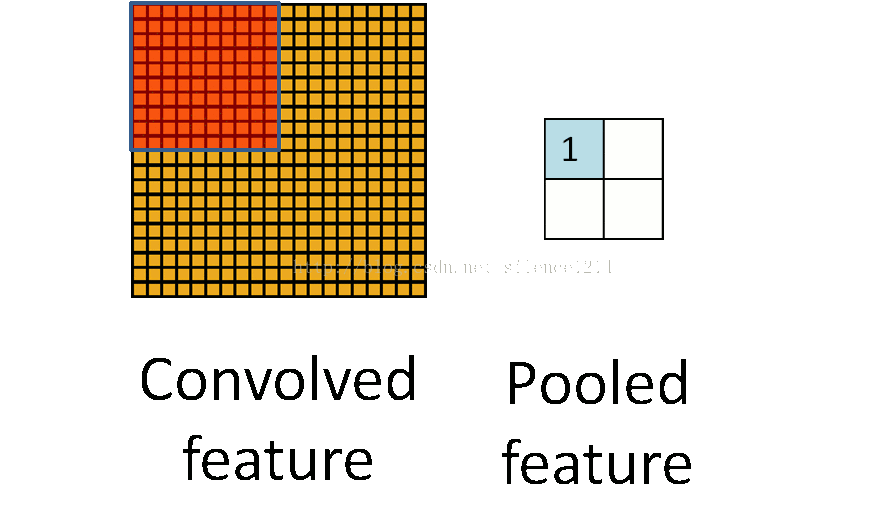3. 卷积层
卷积层是通过张量卷积，或者说是若干个矩阵卷积求和而得到当前层的输出，这和DNN直接进行矩阵乘法有很大区别，那么如何递推相应的δl−1${\delta }^{l-1}$$\delta^{l-1}$呢？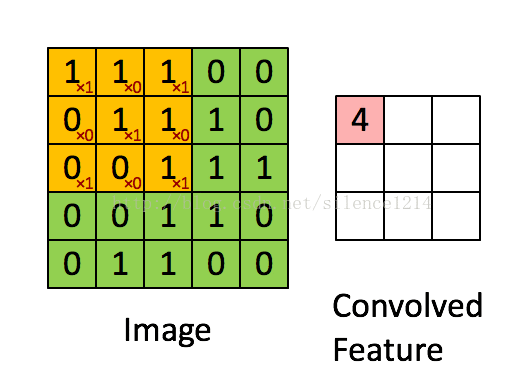4. 反卷积层和BN层
这个日后弄懂再补上来。

池化层的反向传播

池化层没有激活函数可以直接看成用线性激活函数，即σ(z)=z$\sigma \left(z\right)=z$$\sigma(z)=z$，所以σ′(z)=1${\sigma }^{\prime }\left(z\right)=1$$\sigma'(z)=1$。接下来看看池化层如何递推δl${\delta }^{l}$$\delta^l$。
在前向传播时，我们一般使用max或average对输入进行池化，而且池化区域大小已知。反向传播就是要从缩小后的误差δl+1${\delta }^{l+1}$$\delta^{l+1}$，还原池化前较大区域对应的误差δl${\delta }^{l}$$\delta^l$。根据（BP2），δl=((wl+1)Tδl+1)⊙σ′(zl)${\delta }^{l}=\left(\left({w}^{l+1}{\right)}^{T}{\delta }^{l+1}\right)\odot {\sigma }^{\prime }\left({z}^{l}\right)$$\delta^l=((w^{l+1})^T\delta^{l+1})\odot \sigma'(z^l)$，在DNN中wl+1${w}^{l+1}$$w^{l+1}$是已知的，所以我们可以直接通过矩阵乘法将l+1$l+1$$l+1$层的误差映射回l$l$$l$层的误差，但对于池化层，要求(wl+1)Tδl+1$\left({w}^{l+1}{\right)}^{T}{\delta }^{l+1}$$(w^{l+1})^T\delta^{l+1}$就需要一些特殊的操作了。
用一个例子可以很清楚的解释这一过程：假设现在我们是步长为1的2×2$2×2$$2\times 2$池化，4×4$4×4$$4 \times 4$大小的区域经过池化后变为2×2$2×2$$2\times 2$。如果δl${\delta }_{l}$$\delta_l$的第k个子矩阵为：δl+1k=${\delta }_{k}^{l+1}=\left[\begin{array}{cc}2& 8\\ 4& 6\end{array}\right]$\delta_k^{l+1}=\left[ \begin{matrix}2 & 8\\4 & 6 \end{matrix} \right]首先我们要确定δl+1k${\delta }_{k}^{l+1}$$\delta_k^{l+1}$中4个误差值分别和原来4×4$4×4$$4\times 4$大小的哪个子区域所对应，根据前向传播中池化窗口的移动过程，我们可以很轻松的确定2对应左上角2×2$2×2$$2\times 2$的区域，8对应右上角2×2$2×2$$2\times 2$的区域，以此类推。这一步完成之后，我们就要对不同类型的池化进行不同的操作。
如果是max pooling，我们只需要记录前向传播中最大值的位置，然后将误差放回去即可。如果最大值位置分别为2×2$2×2$$2\times 2$的左上，右下，右上，左下，还原后的矩阵为：
(wl+1)Tδl+1=⎡⎣⎢⎢⎢2000004000060800⎤⎦⎥⎥⎥$\left({w}^{l+1}{\right)}^{T}{\delta }^{l+1}=\left[\begin{array}{cccc}2& 0& 0& 0\\ 0& 0& 0& 8\\ 0& 4& 0& 0\\ 0& 0& 6& 0\end{array}\right]$(w^{l+1})^T\delta^{l+1}=\left[ \begin{matrix}2&0&0&0\\0&0&0&8\\0&4&0&0\\0&0&6&0 \end{matrix} \right]
如果是average pooing，我们只需要将池化单元的误差平均值放回原来的子矩阵即可：
(wl+1)Tδl+1=⎡⎣⎢⎢⎢0.50.5110.50.511221.51.5221.51.5⎤⎦⎥⎥⎥$\left({w}^{l+1}{\right)}^{T}{\delta }^{l+1}=\left[\begin{array}{cccc}0.5& 0.5& 2& 2\\ 0.5& 0.5& 2& 2\\ 1& 1& 1.5& 1.5\\ 1& 1& 1.5& 1.5\end{array}\right]$(w^{l+1})^T\delta^{l+1}=\left[ \begin{matrix}0.5&0.5&2&2\\0.5&0.5&2&2\\1&1&1.5&1.5\\1&1&1.5&1.5 \end{matrix} \right]可以发现这其实就是将上一层的误差进行一次池化的逆操作，还是比较容易理解的。
得到了(wl+1)Tδl+1$\left({w}^{l+1}{\right)}^{T}{\delta }^{l+1}$$(w^{l+1})^T\delta^{l+1}$之后就可以利用δl=((wl+1)Tδl+1)⊙σ′(zl)${\delta }^{l}=\left(\left({w}^{l+1}{\right)}^{T}{\delta }^{l+1}\right)\odot {\sigma }^{\prime }\left({z}^{l}\right)$$\delta^l=((w^{l+1})^T\delta^{l+1})\odot \sigma'(z^l)$求得δlk${\delta }_{k}^{l}$$\delta_k^l$了。

卷积层的反向传播

继续回到方程（BP2），δl=((wl+1)Tδl+1)⊙σ′(zl)${\delta }^{l}=\left(\left({w}^{l+1}{\right)}^{T}{\delta }^{l+1}\right)\odot {\sigma }^{\prime }\left({z}^{l}\right)$$\delta^l=((w^{l+1})^T\delta^{l+1})\odot \sigma'(z^l)$，那你可能会问，之前说池化层因为wl+1${w}^{l+1}$$w^{l+1}$无法直接计算，所以需要特殊操作，那么卷积核的参数不是知道吗，岂不是可以直接代入计算了。是带进去计算没错，但是权重矩阵需要旋转180°。为什么呢，下面以一个简单的例子说明。
假设l$l$$l$层的激活输出是一个3×3$3×3$$3\times 3$的矩阵，第l+1$l+1$$l+1$层卷积核Wl+1${W}^{l+1}$$W^{l+1}$是一个2×2$2×2$$2\times 2$的矩阵，卷积步长为1，则输出zl+1${z}^{l+1}$$z^{l+1}$是一个2×2$2×2$$2\times 2$的矩阵。我们简化bl=0${b}^{l}=0$$b^l=0$，则有：zl+1=al∗Wl+1(1)$\begin{array}{}\text{(1)}& {z}^{l+1}={a}^{l}\ast {W}^{l+1}\end{array}$z^{l+1}=a^l*W^{l+1} \tag{1}列出a$a$$a$，W$W$$W$，z$z$$z$的矩阵表达式如下：[z11z21z12z22]=⎡⎣⎢a11a21a31a12a22a32a13a23a33⎤⎦⎥∗[w11w21w12w22](2)$\begin{array}{}\text{(2)}& \left[\begin{array}{cc}{z}_{11}& {z}_{12}\\ {z}_{21}& {z}_{22}\end{array}\right]=\left[\begin{array}{ccc}{a}_{11}& {a}_{12}& {a}_{13}\\ {a}_{21}& {a}_{22}& {a}_{23}\\ {a}_{31}& {a}_{32}& {a}_{33}\end{array}\right]\ast \left[\begin{array}{cc}{w}_{11}& {w}_{12}\\ {w}_{21}& {w}_{22}\end{array}\right]\end{array}$\left[ \begin{matrix} z_{11}&z_{12}\\z_{21}&z_{22}\end{matrix} \right]=\left[ \begin{matrix} a_{11}&a_{12}&a_{13}\\a_{21}&a_{22}&a_{23}\\a_{31}&a_{32}&a_{33}\end{matrix} \right] * \left[ \begin{matrix} w_{11}&w_{12}\\w_{21}&w_{22}\end{matrix} \right] \tag{2}利用卷积的定义，很容易得出：z11=a11w11+a12w12+a21w21+a22w22z12=a12w11+a13w12+a22w21+a23w22z21=a21w11+a22w12+a31w21+a32w22z22=a22w11+a23w12+a32w21+a33w22(3)$\begin{array}{}\text{(3)}& {z}_{11}={a}_{11}{w}_{11}+{a}_{12}{w}_{12}+{a}_{21}{w}_{21}+{a}_{22}{w}_{22}\phantom{\rule{0ex}{0ex}}{z}_{12}={a}_{12}{w}_{11}+{a}_{13}{w}_{12}+{a}_{22}{w}_{21}+{a}_{23}{w}_{22}\phantom{\rule{0ex}{0ex}}{z}_{21}={a}_{21}{w}_{11}+{a}_{22}{w}_{12}+{a}_{31}{w}_{21}+{a}_{32}{w}_{22}\phantom{\rule{0ex}{0ex}}{z}_{22}={a}_{22}{w}_{11}+{a}_{23}{w}_{12}+{a}_{32}{w}_{21}+{a}_{33}{w}_{22}\end{array}$z_{11}=a_{11}w_{11}+a_{12}w_{12}+a_{21}w_{21}+a_{22}w_{22}\\z_{12}=a_{12}w_{11}+a_{13}w_{12}+a_{22}w_{21}+a_{23}w_{22}\\z_{21}=a_{21}w_{11}+a_{22}w_{12}+a_{31}w_{21}+a_{32}w_{22}\\z_{22}=a_{22}w_{11}+a_{23}w_{12}+a_{32}w_{21}+a_{33}w_{22} \tag{3}接下来我们计算∂C∂al$\frac{\mathrm{\partial }C}{\mathrm{\partial }{a}^{l}}$$\frac{\partial C}{\partial a^l}$：∇al=∂C∂al=∂C∂zl+1∂zl+1∂al=δl+1∂zl+1∂al(4)$\begin{array}{}\text{(4)}& \mathrm{\nabla }{a}^{l}=\frac{\mathrm{\partial }C}{\mathrm{\partial }{a}^{l}}=\frac{\mathrm{\partial }C}{\mathrm{\partial }{z}^{l+1}}\frac{\mathrm{\partial }{z}^{l+1}}{\mathrm{\partial }{a}^{l}}={\delta }^{l+1}\frac{\mathrm{\partial }{z}^{l+1}}{\mathrm{\partial }{a}^{l}}\end{array}$\nabla a^l=\frac{\partial C}{\partial a^l}=\frac{\partial C}{\partial z^{l+1}}\frac{\partial z^{l+1}}{\partial a^l}=\delta^{l+1}\frac{\partial z^{l+1}}{\partial a^l} \tag{4}由方程(2)可以得知，∂zl+1∂al$\frac{\mathrm{\partial }{z}^{l+1}}{\mathrm{\partial }{a}^{l}}$$\frac{\partial z^{l+1}}{\partial a^l}$和Wl+1${W}^{l+1}$$W^{l+1}$相关。假设δl+1=[δ11δ21δ12δ22]${\delta }^{l+1}=\left[\begin{array}{cc}{\delta }_{11}& {\delta }_{12}\\ {\delta }_{21}& {\delta }_{22}\end{array}\right]$\delta^{l+1}=\left[ \begin{matrix}\delta_{11} & \delta_{12}\\ \delta_{21} & \delta_{22}\end{matrix} \right]在式（3）的4个等式中，a11${a}_{11}$$a_{11}$只和z11${z}_{11}$$z_{11}$有关（z12,z21,z22${z}_{12},{z}_{21},{z}_{22}$$z_{12},z_{21},z_{22}$表达式中均没有a11${a}_{11}$$a_{11}$），所以∇a11=δl+111∂zl+111∂al11+δl+112∂zl+112∂al11+δl+121∂zl+121∂al11+δl+122∂zl+122∂al11=δ11w11$\mathrm{\nabla }{a}_{11}={\delta }_{11}^{l+1}\frac{\mathrm{\partial }{z}_{11}^{l+1}}{\mathrm{\partial }{a}_{11}^{l}}+{\delta }_{12}^{l+1}\frac{\mathrm{\partial }{z}_{12}^{l+1}}{\mathrm{\partial }{a}_{11}^{l}}+{\delta }_{21}^{l+1}\frac{\mathrm{\partial }{z}_{21}^{l+1}}{\mathrm{\partial }{a}_{11}^{l}}+{\delta }_{22}^{l+1}\frac{\mathrm{\partial }{z}_{22}^{l+1}}{\mathrm{\partial }{a}_{11}^{l}}={\delta }_{11}{w}_{11}$\nabla a_{11}=\delta_{11}^{l+1}\frac{\partial z_{11}^{l+1}}{\partial a_{11}^l}+\delta_{12}^{l+1}\frac{\partial z_{12}^{l+1}}{\partial a_{11}^l}+\delta_{21}^{l+1}\frac{\partial z_{21}^{l+1}}{\partial a_{11}^l}+\delta_{22}^{l+1}\frac{\partial z_{22}^{l+1}}{\partial a_{11}^l}=\delta_{11} w_{11}同理可以得到其他8个∇a$\mathrm{\nabla }a$$\nabla a$：∇a12=δ11w12+δ12w11∇a13=δ12w12∇a21=δ11w21+δ21w11∇a22=δ11w22+δ12w21+δ21w12+δ22w11∇a23=δ12w22+δ22w12∇a31=δ21w21∇a32=δ21w22+δ22w21∇a33=δ22w22$\mathrm{\nabla }{a}_{12}={\delta }_{11}{w}_{12}+{\delta }_{12}{w}_{11}\phantom{\rule{0ex}{0ex}}\mathrm{\nabla }{a}_{13}={\delta }_{12}{w}_{12}\phantom{\rule{0ex}{0ex}}\mathrm{\nabla }{a}_{21}={\delta }_{11}{w}_{21}+{\delta }_{21}{w}_{11}\phantom{\rule{0ex}{0ex}}\mathrm{\nabla }{a}_{22}={\delta }_{11}{w}_{22}+{\delta }_{12}{w}_{21}+{\delta }_{21}{w}_{12}+{\delta }_{22}{w}_{11}\phantom{\rule{0ex}{0ex}}\mathrm{\nabla }{a}_{23}={\delta }_{12}{w}_{22}+{\delta }_{22}{w}_{12}\phantom{\rule{0ex}{0ex}}\mathrm{\nabla }{a}_{31}={\delta }_{21}{w}_{21}\phantom{\rule{0ex}{0ex}}\mathrm{\nabla }{a}_{32}={\delta }_{21}{w}_{22}+{\delta }_{22}{w}_{21}\phantom{\rule{0ex}{0ex}}\mathrm{\nabla }{a}_{33}={\delta }_{22}{w}_{22}$\nabla a_{12}=\delta_{11}w_{12}+\delta_{12}w_{11}\\ \nabla a_{13}=\delta_{12}w_{12}\\ \nabla a_{21}=\delta_{11}w_{21}+\delta_{21}w_{11}\\ \nabla a_{22}=\delta_{11}w_{22}+\delta_{12}w_{21}+\delta_{21}w_{12}+\delta_{22}w_{11}\\ \nabla a_{23}=\delta_{12}w_{22}+\delta_{22}w_{12}\\ \nabla a_{31}=\delta_{21}w_{21}\\ \nabla a_{32}=\delta_{21}w_{22}+\delta_{22}w_{21}\\ \nabla a_{33}=\delta_{22}w_{22}其实上面的9个式子可以用一个矩阵卷积的形式统一表示：
⎡⎣⎢∇a11∇a21∇a31∇a12∇a22∇a32∇a13∇a23∇a33⎤⎦⎥=⎡⎣⎢⎢⎢00000δ11δ2100δ12δ2200000⎤⎦⎥⎥⎥∗[w22w12w21w11](5)$\begin{array}{}\text{(5)}& \left[\begin{array}{ccc}\mathrm{\nabla }{a}_{11}& \mathrm{\nabla }{a}_{12}& \mathrm{\nabla }{a}_{13}\\ \mathrm{\nabla }{a}_{21}& \mathrm{\nabla }{a}_{22}& \mathrm{\nabla }{a}_{23}\\ \mathrm{\nabla }{a}_{31}& \mathrm{\nabla }{a}_{32}& \mathrm{\nabla }{a}_{33}\end{array}\right]=\left[\begin{array}{cccc}0& 0& 0& 0\\ 0& {\delta }_{11}& {\delta }_{12}& 0\\ 0& {\delta }_{21}& {\delta }_{22}& 0\\ 0& 0& 0& 0\end{array}\right]\ast \left[\begin{array}{cc}{w}_{22}& {w}_{21}\\ {w}_{12}& {w}_{11}\end{array}\right]\end{array}$\left[ \begin{matrix} \nabla a_{11}&\nabla a_{12}&\nabla a_{13}\\\nabla a_{21}&\nabla a_{22}&\nabla a_{23}\\\nabla a_{31}&\nabla a_{32}&\nabla a_{33}\end{matrix} \right]=\left[ \begin{matrix}0&0&0&0\\ 0&\delta_{11} & \delta_{12}&0\\ 0&\delta_{21} & \delta_{22}&0\\0&0&0&0\end{matrix} \right] * \left[ \begin{matrix} w_{22}&w_{21}\\w_{12}&w_{11}\end{matrix} \right] \tag{5}
为了符合梯度计算，我们在误差矩阵周围填充了一圈0，此时我们将卷积核翻转后和反向传播的梯度误差进行卷积，就得到了前一次的梯度误差，然后用（BP2）就可以得到上一层的误差。卷积层的（BP2）形式如下：δl=(δl+1∗rot180(wl+1))⊙σ′(zl)${\delta }^{l}=\left({\delta }^{l+1}\ast rot180\left({w}^{l+1}\right)\right)\odot {\sigma }^{\prime }\left({z}^{l}\right)$\delta^l=(\delta^{l+1} * rot180(w^{l+1}))\odot \sigma'(z^l)
还需要注意的是，在利用（BP4）推导该层权重的梯度∂C∂wl$\frac{\mathrm{\partial }C}{\mathrm{\partial }{w}^{l}}$$\frac{\partial C}{\partial w^l}$时，也需要进行一个旋转180°的操作：∂C∂wl=∂C∂zl∂zL∂wl=δl∂zL∂wl=δl∗rot180(al−1)$\frac{\mathrm{\partial }C}{\mathrm{\partial }{w}^{l}}=\frac{\mathrm{\partial }C}{\mathrm{\partial }{z}^{l}}\frac{\mathrm{\partial }{z}^{L}}{\mathrm{\partial }{w}^{l}}={\delta }^{l}\frac{\mathrm{\partial }{z}^{L}}{\mathrm{\partial }{w}^{l}}={\delta }^{l}\ast rot180\left({a}^{l-1}\right)$\frac{\partial C}{\partial w^l}=\frac{\partial C}{\partial z^l}\frac{\partial z^L}{\partial w^l}=\delta^l\frac{\partial z^L}{\partial w^l}=\delta^l*rot180(a^{l-1})
对于偏置b$b$$b$则有些特殊，因为δl${\delta }^{l}$$\delta^l$是3维张量，而bl${b}^{l}$$b^l$只是一个一维向量，不能像DNN中那样直接∂C∂bl=δl$\frac{\mathrm{\partial }C}{\mathrm{\partial }{b}^{l}}={\delta }^{l}$$\frac{\partial C}{\partial b^l}=\delta^l$，通常是将δl${\delta }^{l}$$\delta^l$的各个子矩阵分别求和，得到一个误差向量，即bl${b}^{l}$$b^l$的梯度：
∂C∂bl=∑u,v(δl)u,v$\frac{\mathrm{\partial }C}{\mathrm{\partial }{b}^{l}}=\sum _{u,v}\left({\delta }^{l}{\right)}_{u,v}$\frac{\partial C}{\partial b^l}=\sum_{u,v}(\delta^l)_{u,v}

总结

虽然CNN的反向传播和DNN有所不同，但本质上还是4个核心公式的变形，思路是一样的。
展开全文卷积神经网络
• 虽然理解了cnn如何运作，但是在反向传播 （包括在softmax逻辑回归和前一层隐含层之间 卷积层和隐含层之间 卷积层和卷积层之间） 如何反向传播 虽无法理解是卷积层参数大概是卷积核，那后一层参数如何对...python
• http://courses.cs.tau.ac.il/Caffe_workshop/Bootcamp/pdf_lectures/Lecture%203%20CNN%20-%20backpropagation.pdf http://courses.cs.tau.ac.il/Caffe_workshop/Bootcamp/pdf_lectures/ ...
http://courses.cs.tau.ac.il/Caffe_workshop/Bootcamp/pdf_lectures/Lecture%203%20CNN%20-%20backpropagation.pdf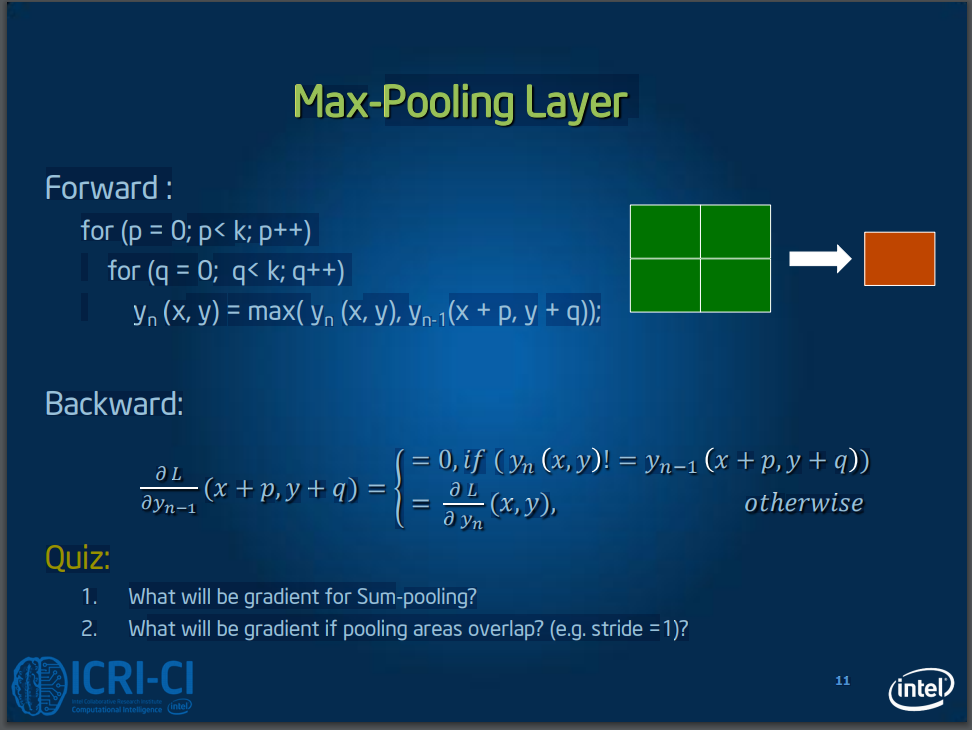http://courses.cs.tau.ac.il/Caffe_workshop/Bootcamp/pdf_lectures/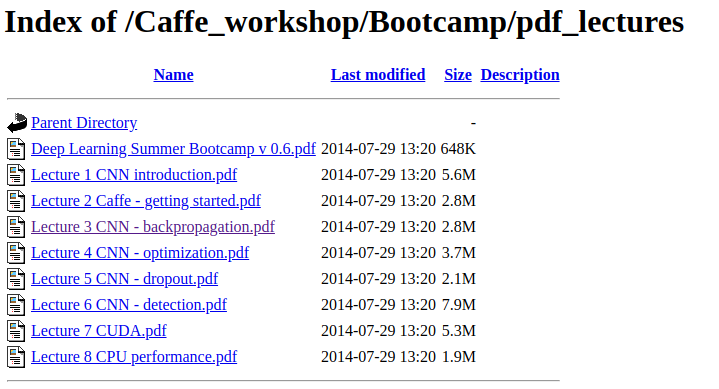https://medium.com/@pavisj/convolutions-and-backpropagations-46026a8f5d2c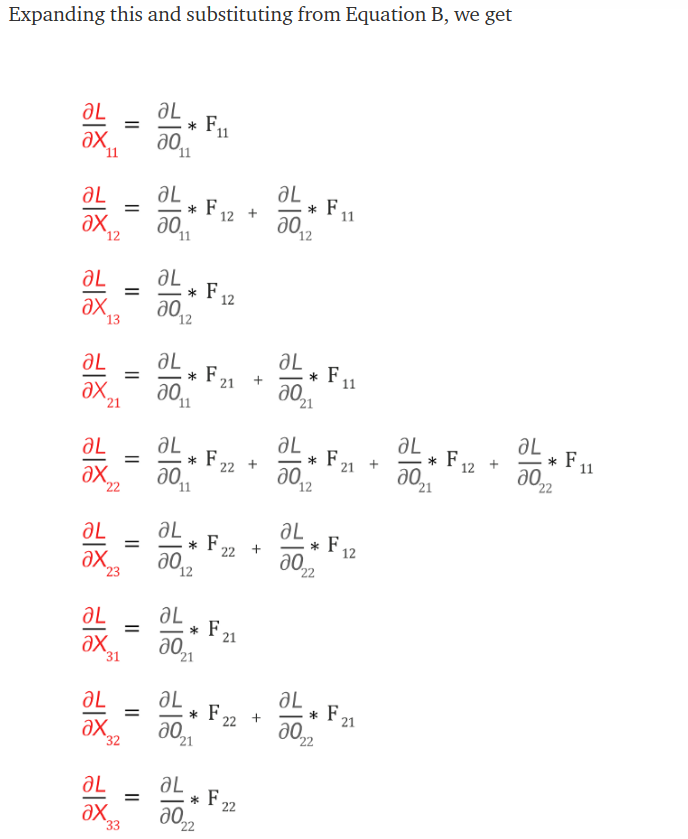http://cbelwal.blogspot.com/2018/05/part-i-backpropagation-mechanics-for.html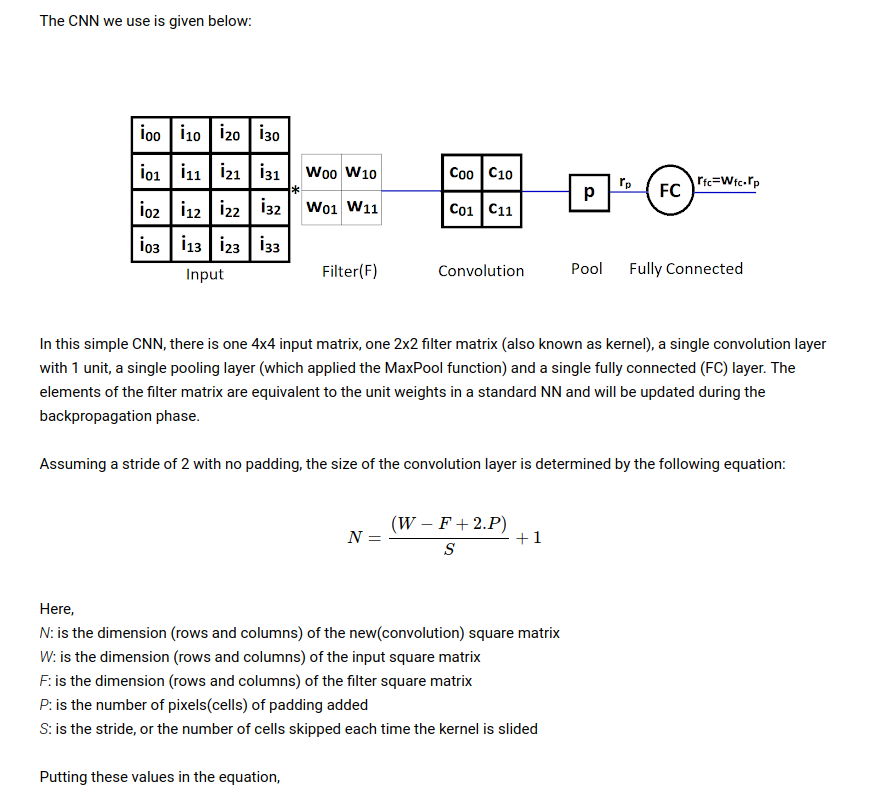https://jianfengwang.files.wordpress.com/2015/07/forwardandbackwardpropagationofconvolutionallayer.pdf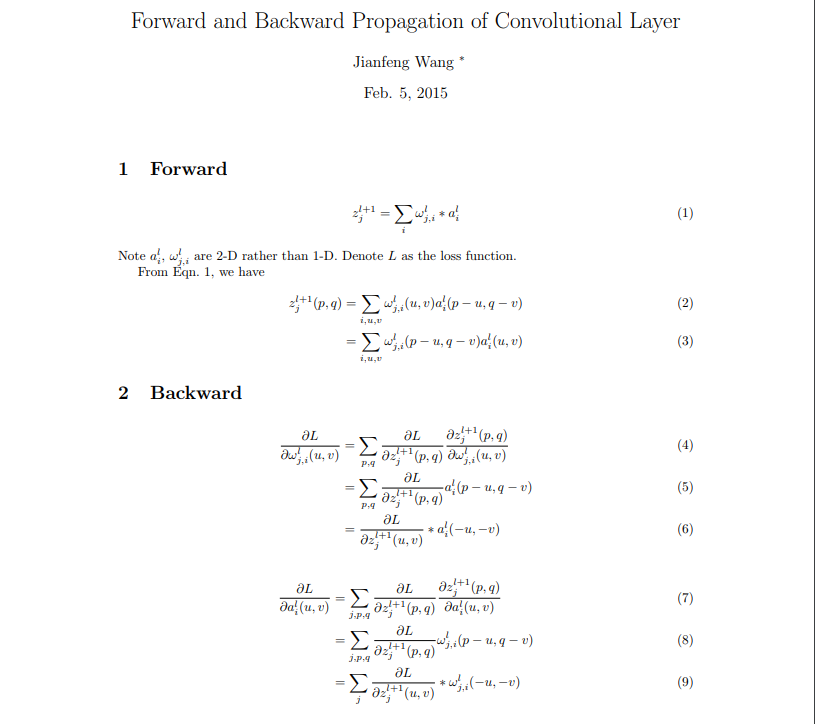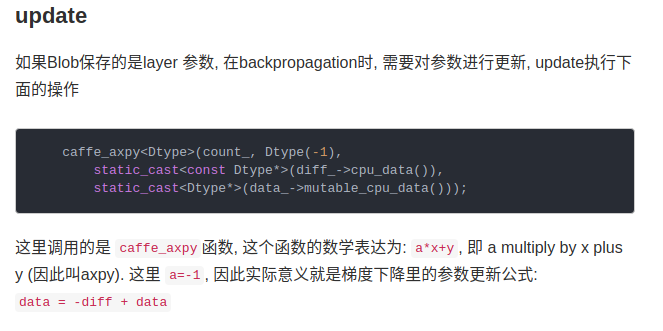展开全文• ## CNN的反向传播推导

千次阅读 2019-01-21 17:48:29
（1）局部感受野：在全连接网络中，输入被描绘成纵向排列神经元，但是在卷积网络中我们把它看成28x28方形： 输入神经元一小片区域会被连接到下一层隐层，这个区域被称为局部感受野，然后在输入图像中移动...
• 本文主要是通过阅读相关论文和一些辅助的博文来理解卷积神经网络里面的反向传播算法；相关的论文可以查看Notes on Convolutional Neural Networks, Jake Bouvrie 准备工作 首先呢，我们还是先回顾下在深度学习...
• 上一篇文章介绍了DNN的反向传播，对DNN不清楚的可以去看看，CNN在DNN的基础上进行解释。 一、回顾DNN的反向传播 上一篇DNN反向传播过程得出结论： 1、计算每一层的W、b的梯度，需要计算出 2、然后每一层的W的...卷积层
• 与深度神经网络DNN的反向传播算法一致，辅助变量： {δL=∂J∂zL=∂J∂aL⊙σ′(zL)δl=(Wl+1)Tδl+1⊙σ′(zl)\left\{\begin{aligned} &amp;\delta^L = \frac{\partial J}{\partial z^L} = \frac{\partial ...卷积神经网络 卷积层 池化层
• 上一篇文章介绍了DNN的反向传播，对DNN不清楚的可以去看看，CNN在DNN的基础上进行解释。 一、回顾DNN的反向传播 上一篇DNN反向传播过程得出结论： 1、计算每一层的W、b的梯度，需要计算出 2、然后每一层的W的梯度和b...
• 在卷积神经网络(CNN)前向传播算法中，我们对CNN的前向传播算法做了总结，基于CNN前向传播算法的基础，我们下面就对CNN的反向传播算法做一个总结。在阅读本文前，建议先研究DNN的反向传播算法：深度神经网络（DNN）...
• 本文详细讲解dnn和cnn的反向传播流程。其实无论是dnn还是cnn、pool层，最重要的是，我们要弄清楚反向传播的四个公式，弄清楚他们，我们就明白整个网络是如何运作和优化的，4个核心公式分别是： 1、输出层的误差...深度学习 算法 卷积 神经网络
• 　在卷积神经网络(CNN)前向传播算法中，我们对CNN的前向传播算法做了总结，基于CNN前向传播算法的基础，我们下面就对CNN的反向传播算法做一个总结。在阅读本文前，建议先研究DNN的反向传播算法：深度神经网络（DNN）...
•  本文首先简单介绍CNN的结构，并不作详细介绍，若需要了解推荐看...本文只要讲解CNN的反向传播CNN的反向传播，其实并不是大多所说的和全连接的BP类似，CNN的全连接部分的BP是与它相同，但是CNN中卷积--池化、池
• CNN反向传播的不同之处： 首先要注意是，一般神经网络中每一层输入输出a,z都只是一个向量，而CNNa,z是一个三维张量，即由若干个输入子矩阵组成。其次： 池化层没有激活函数。这个问题倒比较好解决，我们...
• 在卷积神经网络(CNN)前向传播算法中，我们对CNN的前向传播算法做了总结，基于CNN前向传播算法的基础，我们下面就对CNN的反向传播算法做一个总结。在阅读本文前，建议先研究DNN的反向传播算法：深度神经网络（DNN）...
• 学习CNN的反向传播算法之前最后先弄明白全连接网络的反向传播算法。裂墙推荐这个篇博客神经网络BP反向传播算法原理和详细推导流程，保证博到病除。 CNN 中的卷积操作则不再是全连接的形式，因此 CNN 的 BP 算法需要...深度学习...